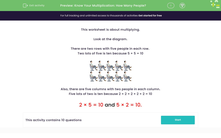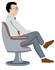# Multiply simple numbers

In this worksheet, students answer questions that require them to use their multiplication skills.Key stage:  KS 2

Curriculum topic:   Number: Multiplication and Division

Curriculum subtopic:   Use Multiplication/Division Facts (3, 4 and 8)

Difficulty level:#### Worksheet Overview

In this activity, we will be solving multiplication problems using pictures to help.

Look at the diagram. It shows a multiplication problem.

There are two rows with five people in each row.Also, there are five columns with two people in each column.

We could write 2 different multiplication calculations for this picture.

2 × 5 = 10

5 × 2 = 10

It doesn't matter which way round we write the numbers, as multiplication is commutative.

This means it can be done in any order.

Let's try an example question together.

Example

There are 3 rows.

There are 2 seats in each row.How many seats are there?

We could write 2 different multiplication calculations for this picture.

2 × 3 = 6

3 × 2 = 6

Now it's your turn to complete some questions like this.

### What is EdPlace?

We're your National Curriculum aligned online education content provider helping each child succeed in English, maths and science from year 1 to GCSE. With an EdPlace account you’ll be able to track and measure progress, helping each child achieve their best. We build confidence and attainment by personalising each child’s learning at a level that suits them.

Get started